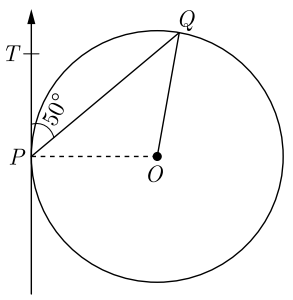Friday, October 22, 2021
Home > CBSE Class 10 > MCQ Questions for Class 10 Maths Chapter 10 Circles with Answer Keys

# MCQ Questions for Class 10 Maths Chapter 10 Circles with Answer KeysHi students, Welcome to AMBiPi (Amans Maths Blogs). In this article, you will get MCQ Questions for Class 10 Maths Chapter 10 Circles with Answer Keys PDF. You can download this PDF and save it in your mobile device or laptop etc.

MCQ Questions for Class 10 Maths Chapter 10 Circles Question No 1:

From an external point Q, the length of tangent to a circle is 12 cm and the distance of Q from the centre of circle is 13 cm. The radius of circle (in cm) is

Option A : 10

Option B : 12

Option C : 5

Option D : 7

Option B : 12

MCQ Questions for Class 10 Maths Chapter 10 Circles Question No 2:

Two circle touch each other externally at C and AB is a common tangent to the circles. Then, ∠ACB =

Option A : 60 degrees

Option B : 45 degrees

Option C : 30 degrees

Option D : 90 degrees

Option D : 90 degrees

MCQ Questions for Class 10 Maths Chapter 10 Circles Question No 3:

QP is a tangent to a circle with centre O at a point P on the circle. If triangle OPQ is isosceles, then ∠OQR

Option A : 30 degrees

Option B : 45 degrees

Option C : 60 degrees

Option D : 90 degrees

Option B : 45 degrees

MCQ Questions for Class 10 Maths Chapter 10 Circles Question No 4:

If TP and TQ are two tangents to a circle with centre O so that ∠POQ = 110°, then, ∠PTQ is equal to

Option A : 60 degrees

Option B : 70 degrees

Option C : 80 degrees

Option D : 90 degrees

Option B : 70 degrees

MCQ Questions for Class 10 Maths Chapter 10 Circles Question No 5:

A chord of a circle of radius 10 cm, subtends a right angle at its centre. The length of the chord (in cm) is

Option A : 5/√2 cm

Option B : 5√2 cm

Option C : 10√2 cm

Option D : 10√2 cm

Option C : 10√2 m

MCQ Questions for Class 10 Maths Chapter 10 Circles Question No 6:

Tangents from an external point to a circle are

Option A : equal

Option B : not equal

Option C : parallel

Option D : perpendicular

Option A : equal

MCQ Questions for Class 10 Maths Chapter 10 Circles Question No 7:

In figure, O is the centre of circle. PQ is a chord and PT is tangent at P which makes an angle of 50c with PQ, then ∠POQ isOption A : 130o

Option B : 90o

Option C : 100o

Option D : 75o

Option C : 100o

MCQ Questions for Class 10 Maths Chapter 10 Circles Question No 8:

Two parallel lines touch the circle at points A and B respectively. If area of the circle is 25 cm2, then AB is equal to

Option A : 5 cm

Option B : 8 cm

Option C : 10 cm

Option D : 25 cm

Option C : 10 cm

MCQ Questions for Class 10 Maths Chapter 10 Circles Question No 9:

In figure, AP, AQ and BC are tangents of the circle with centre O. If AB = 5 cm, AC = 6 cm and BC = 4 cm, then the length of AP (in cm) isOption A : 15

Option B : 10

Option C : 9

Option D : 7.5

Option D : 7.5

MCQ Questions for Class 10 Maths Chapter 10 Circles Question No 10:

A line through point of contact and passing through centre of circle is known as

Option A : tangent

Option B : chord

Option C : normal

Option D : secant